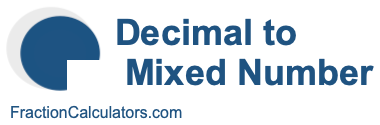Decimal to Mixed Number CalculatorWelcome to our Decimal to Mixed Number Calculator. This calculator can convert any decimal number to a mixed number. In other words, it can convert a decimal number to a whole number (W) and a fraction with a numerator (N) and a denominator (D). Here is the illustration of what we are converting:

Decimal ->> Mixed Number
Decimal ->> W N/D

Before we continue, and in case you are wondering, note that mixed number is the same as mixed fraction. Without further ado, please enter the decimal number that you want to convert to a mixed number.

What is  as a mixed number?

Here are some examples of what our Decimal to Mixed Number Calculator can calculate and convert for you.

What is 2.5 as a mixed number?

What is 8.21 as a mixed number?

What is 5.37 as a mixed number?

What is 2.25 as a mixed number?

What is 3.5 as a mixed number?

What is 5.75 as a mixed number?

What is 4.75 as a mixed number?

What is 5.25 as a mixed number?